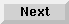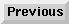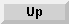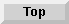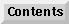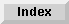5 Operators and Functions

## Relational Operators

The relational operators are:
Operators Operations Examples
<less thanA < B
>greater thanA > B
<=less than or equal toA <= B
>=greater than or equal toA >= B
=equalsA = B
<> or #not equal toA <> B

When relational operators are used in a numeric expression, the value 1 is returned if the relation is found to be true; the value 0 is returned if the relation is false. For instance, A=B is evaluated as 1 if A and B are equal in value, 0 if they are not equal. If A=1, B=2, and C=3, then (A*B)<(A-C/6) is evaluated as 0 (false) because A times B equals 2, which is not less than 0.5 (the result of (A-C/6)).

Here is a sample program:

```10   INPUT "ENTER THREE VALUES:";A,B,C
20   Logic=(A*B<C)
30   If Logic THEN
40       PRINT "(A*B<C) is TRUE and has a value of ";Logic
50   ELSE
60       PRINT "(A*B<C) is FALSE and has a value of ";Logic
70   END IF
80   END
```
Entering the values 3, 4, and 5 results in:

```(A*B<C) is FALSE and has a value of 0
```
Notice that the IF expression is true for any non-zero value.

Relational operators are also used to compare strings. Strings are compared according to their associated numeric values in the ASCII code (see page 391 ). The strings are compared character-by-character until a difference is found or until the end of a string is reached. If the ends of both strings are found at the same time, the strings are equal. If the end of one string is reached first, however, then that string is an initial substring of the other, and is considered to be less than the longer string. For example, all the following expressions are true:

```"AB" < "ABC"   "AB" is an initial substring of "ABC".

"AB" = "AB"     Both strings are exactly equal.

"B" > "ABC"  "B" has a higher numeric equivalent than "A" in the ASCII code.

"AB\$" < "AB*" "\$" has a lower numeric equivalent than "*" in the ASCII code.
```
The null string ("") is always less than every other string and equal only to another null string. More information on substrings is given in page 73 . Relational operators are also usable with foreign-language keyboards, but results may not match ASCII values.

Eloquence Language Manual - 19 DEC 2002